Math resources Geometry Area

Area of a rhombus

# Area of a rhombus

Here you will learn about finding the area of a rhombus, including how to find missing dimensions and convert units to find area.

Students first learn how to find the area of quadrilaterals such as rectangles and squares in the 3 rd grade and continue to build upon that knowledge as they progress into middle school.

## What is the area of a rhombus?

The area of a rhombus is the amount of space inside the rhombus. Like with other 2D shapes, the area is measured in units squares \left(\mathrm{cm}^2, \mathrm{~m}^2, \mathrm{~mm}^2 \text { etc }\right). A rhombus is a special type of parallelogram that has four sides of equal length.

Since a rhombus is a parallelogram, you can find the area the same way you find the area of a parallelogram.

{Area} = length \: of \: the \: base \times length \: of \: the \: height

{A} = b \times h

For example, let’s find the area of the rhombus with the given dimensions.

The height of the rhombus is 8{~cm} and the base of the rhombus is 10{~cm}.

Using the formula:

\begin{aligned} & \text {Area }=\text { base } \times \text { height } \\\\ & h=8 \\\\ & b=10 \\\\ & \text {Area }=8 \times 10 \\\\ & \text {Area }=80 \end{aligned}

The area of the rhombus is 80 \mathrm{~cm}^2.

Like parallelograms, rhombuses have diagonals that bisect each other. However, the diagonals of a rhombus also form right angles because they are perpendicular.

You can find the area of a rhombus using diagonals.

For example, find the area of the rhombus with the given dimensions.

A rhombus is a parallelogram, so the diagonals are segment bisectors meaning they cut each other into two equal halves.

Each of the four triangles is equal. The base length is 6{~cm}, and the height length is 8{~cm}. This can also be interpreted as the base length as 8{~cm} and the height length as 6{~cm}.

The area of a triangle is A = \cfrac{1}{2} \times b \times h

\begin{aligned} & A=\cfrac{1}{2} \times b \times h \\\\ & b=6 \\\\ & h=8 \\\\ & A=\frac{1}{2} \times 6 \times 8 \\\\ & A=24 \end{aligned}

The area of one of the triangles is 24. So the area of the entire rhombus is, 24+24+24+24=96 \text { or } 4(24)=96

The area of the rhombus is 96 \mathrm{~cm}^2.

In high school, you will learn how to find the area of a rhombus using the area of rhombus formula which is also used to find the area of any quadrilateral with perpendicular diagonals.

\text { Area of rhombus }=\frac{1}{2} \times \text { diagonal } 1 \times \text { diagonal } 2

### What is the area of a rhombus?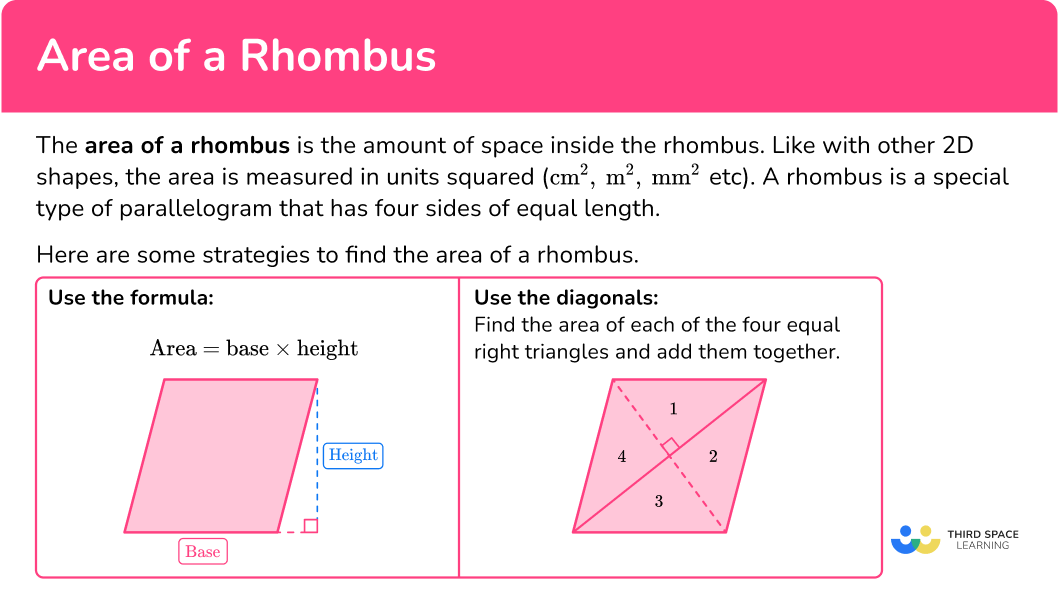## Common Core State Standards

How does this relate to 6 th grade math?

• Grade 6 – Geometry (6.G.A.1)
Find the area of right triangles, other triangles, special quadrilaterals, and polygons by composing into rectangles or decomposing into triangles and other shapes; apply these techniques in the context of solving real-world and mathematical problems.

## How to find the area of a rhombus

In order to find the area of a rhombus:

1. Identify the given dimensions.
2. Substitute the given values into the formula.

## Area of a rhombus examples

### Example 1: finding area given the base and height

Find the area of the rhombus below:

1. Identify the given dimensions.

The height of the rhombus is 4.2 \mathrm{~cm}.

Since a rhombus has four congruent sides, the base of the rhombus is 6.8 \mathrm{~cm}.

2Substitute the given values into the formula.

\begin{aligned} & \text {Area }=\text { base } \times \text { height } \\\\ & \text {Area }=6.8 \times 4.2 \\\\ & \text {Area }=28.56 \end{aligned}

The area of the rhombus is 28.56 \mathrm{~cm}^2.

### Example 2: find the height of the rhombus

The area of the rhombus is 120 \mathrm{~in}^2 and the side length of the rhombus is 12 \text { inches}.

Find the height of the rhombus.

The area of the rhombus is 120 \mathrm{~in}^2 and the base of the rhombus is the same as the side length, which is 12 \mathrm{~in}.

\begin{aligned} & \text {Area }=\text { base } \times \text { height } \\\\ & 120=12 \times \text { height } \\\\ & 120=12 h \\\\ & 120 \div 12=12 \div 12 \mathrm{~h} \\\\ & 10=h \end{aligned}

The height of the rhombus is 10 \text{ inches}.

### Example 3: area of rhombus using diagonals

Find the area of a rhombus where AC=4 \mathrm{~m} and BD=12 \mathrm{~m}.

The diagonals of the rhombus are perpendicular (form right angles) and since a rhombus is a parallelogram, the diagonals also bisect each other. So, AC and BD get divided into two equal halves.

\begin{aligned} & 4 \div 2=2 \\\\ & 12 \div 2=6 \end{aligned}

The diagonals of the rhombus create four congruent (equal) triangles that have a height of 2{~m} and base of 6{~m}. (This can also be interpreted as a height of 6{~m} and base of 2{~m}.

\text{Area of a triangle } =\cfrac{1}{2} \, \times base \times height

\text{Area of triangle }=\cfrac{1}{2} \, \times 6 \times 2

\text{Area of triangle }=6

The area of each triangle is 6 \mathrm{~m}^2.

There are four equal triangles with an area of 6 \mathrm{~m}^2.

So the area of the rhombus is:

\begin{aligned} & \text {Area of rhombus }=6+6+6+6 \text { or } 4(6) \\\\ & \text {Area of rhombus }=24 \mathrm{~m}^2 \end{aligned}

In high school, you will learn to use the area formula for a quadrilateral with perpendicular diagonals.

\begin{aligned} & \text {Area }=\cfrac{1}{2} \times \text { diagonal } 1 \times \text { diagonal } 2 \\\\ & \text {Area }=\cfrac{1}{2} \times 4 \times 12 \\\\ & \text {Area }=24 \mathrm{~m}^2 \end{aligned}

### Example 4: find area convert dimensions

The base of the rhombus is 13.2{~cm}.

The height of the rhombus is 0.114{~m}.

Notice the dimensions are in different units. Before finding the area, make sure the units of the dimensions are the same.

Changing the height which is in meters to centimeters.

\begin{aligned} & 0.114 \times 100=11.4 \\\\ & 0.114 \mathrm{~m}=11.4 \mathrm{~cm} \end{aligned}

\text{Area of a rhombus } = base \times height

\text{Area of a rhombus } =13.2 \times 11.4

\text{Area of a rhombus } =150.48

The area of the rhombus is 150.48 \mathrm{~cm}^2.

### Example 5: word problem

Daniel has a rhombus shaped wooden sign for school spirit week that has an area of 840 \mathrm{~in}^2. He wants to figure out the length of the shorter diagonal in order to make a wooden rod to hang it on. He calculated longer diagonal to be 80 \text { inches}. What is the length of the shorter diagonal?

The diagonals of the rhombus are perpendicular to each other and bisect each other (cut each other into two equal halves).

The longer diagonal is 80 inches, so 80 \div 2=40

Each half of the longer diagonal is 40 inches. The diagonals of a rhombus also divide the rhombus into four congruent triangles that have equal areas.

Since the area of the rhombus is 840 \mathrm{~in}^2 , by dividing the area by 4, you can find the area of one of those triangles.

840 \div 4=210

210 \mathrm{~in}^2 is the area of one of the triangles.

Use the area of one triangle to find half of the length of the shorter diagonal.

\begin{aligned} & \text {Area of triangle }=\frac{1}{2} \times b \times h \\\\ & 210=\cfrac{1}{2} \times 40 \times h \\\\ & 210=20 h \\\\ & 210 \div 20=20 \div 20 h \\\\ & 10.5=h \end{aligned}

The height of one of the triangles is 10.5{~inches}, which is half the length of one of the diagonals.

So, the entire length of the shorter diagonal is 2 \times 10.5=21

The shorter diagonal length is 21{~inches}.

Daniel needs a 21{~inch} length wooden rod.

### Example 6: find perimeter of a rhombus given the area

Find the perimeter of the rhombus with an area of 2,150 \mathrm{~cm}^2 and a height of 43 \mathrm{~cm}.

The height of the rhombus is 43 \mathrm{~cm} and the area is 2,150 \mathrm{~cm}^2.

Using the formula:

Area = base \times height

\begin{aligned} & 2150=\text { base } \times 43 \\\\ & 2150=b \times 43 \\\\ & 2150 \div 43=b \times 43 \div 43 \\\\ & 50=b \end{aligned}

The base of the rhombus is 50{~cm}. Since each side of a rhombus is equal in length, each side of the rhombus is 50{~cm}.

The perimeter can be found by adding up the side lengths.

\begin{aligned} & \text {Perimeter }=50+50+50+50 \\\\ & \text {Perimeter }=200 \end{aligned}

The perimeter of the rhombus is 200{~cm}.

### Teaching tips for area of rhombus

• Teach the conceptual understanding of formulas, such as \text { Area }=\text { base} \times \text { height} by doing active learning activities in class so that students understand what the formula means.

• Infuse learning activities that provide students with an opportunity to explore alternate ways of finding the area of a rhombus, such as dividing it into two or four equal triangles.

• Use digital platforms such as Desmos so students can investigate strategies to find the area of a rhombus.

• Although worksheets provide an opportunity for students to practice skills, using digital platforms that focus on game play is more effective in engaging students.

### Easy mistakes to make

• Forgetting that area is a square unit of measurement
After calculating the area of a 2D shape, students often forget to include the square unit. Area is a two-dimensional measurement, for example,

\begin{aligned} & \text {Area }=(6 \mathrm{~cm}) \times(4 \mathrm{~cm}) \\ & \text {Area }=24 \mathrm{~cm}^2 \end{aligned}

• Forgetting to convert measures to a common unit
Before finding the area of the rhombus or any 2D shape, first make sure all the units are the same. For example, if different units are given ( For example, length = 4{~m} and width = 3{~cm}) , then you must convert them either both to cm or both to m.

• Forgetting that a rhombus is a special parallelogram
Rhombuses have the properties of parallelograms. Therefore, to find the area of a rhombus you can use the formula for finding the area of a parallelogram, \text { Area }=\text { base } \times \text { height.}

### Practice area of a rhombus questions

1) Find the area of the rhombus below: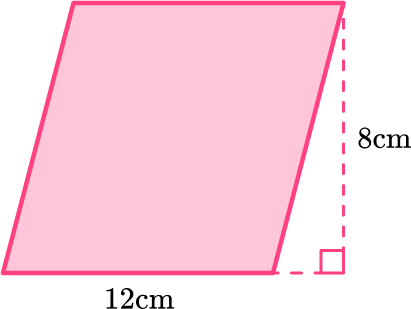96 \mathrm{~cm}96 \mathrm{~cm}^248 \mathrm{~cm}48 \mathrm{~cm}^2A rhombus is a parallelogram so you can use the formula,

\begin{aligned} & \text {Area }=\text { base } \times \text { height } \\\\ & \text {Area }=12 \times 8 \\\\ & \text {Area }=96 \end{aligned}

The area of the rhombus is 96 \mathrm{~cm}^2.

2) The area of the rhombus is 94.32 \mathrm{~in}^2. Find the side length of the rhombus.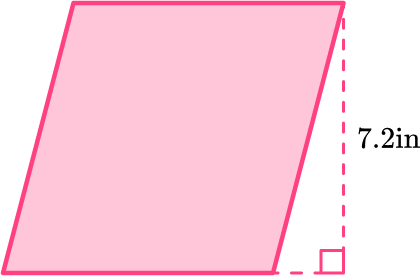13 \text { inches }12 \text { inches}{}^2\text { 13. } 1 \text { inches}\text { 13. } 1 \text { inches}{}^2Substitute known values into the equation, \text { Area }=\text { base } \times \text { height }

\begin{aligned} & \text {Area }=\text { base } \times \text { height } \\\\ & 94.32=\text { base } \times 7.2 \\\\ & 94.32=b \times 7.2 \\\\ & 94.32 \div 7.2=b \times 7.2 \div 7.2 \\\\ & 13.1=b \end{aligned}

The base equals 13.1 inches which means each side is equal to 13.1 inches.

3) Find the area of the rhombus with given diagonal lengths.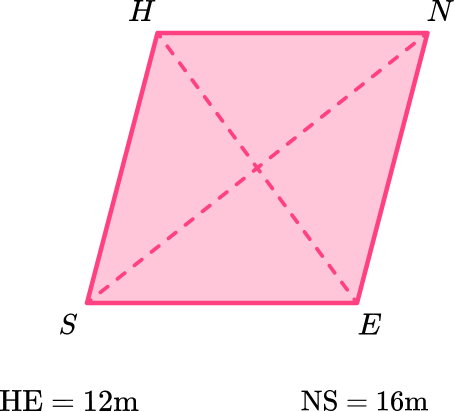192 \mathrm{~m}192 \mathrm{~m}^296 \mathrm{~m}96 \mathrm{~m}^2The diagonals of the rhombus are perpendicular and bisect each other (cut each other into two equal halves). The diagonals also divide the rhombus into four equal triangles with equal areas.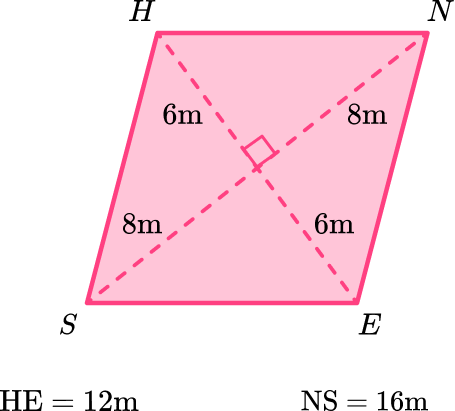Area \: of \: triangle =\cfrac{1}{2} \times base \times height

Area \: of \: triangle =\cfrac{1}{2} \times 6 \times 8

Area \: of \: triangle =24 \mathrm{~m}

If one of the triangles has an area of 24{~m}, then all four triangles have an area of 24{~m}. So the area of the rhombus is 24+24+24+24=96 or 4(24)=96.

Area of the rhombus is 96{~m}^2.

4) Find the area of the rhombus with the given dimensions.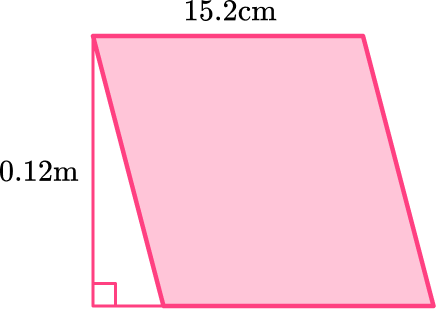182.4 \mathrm{~cm}^21.824 \mathrm{~m}^2182 \mathrm{~m}1.824 \mathrm{~cm}^2The dimensions are given in different units. First, change them to the same unit. There are 100{~cm} in 1 meter so, 0.12 \times 100=12 \mathrm{~cm}.

Substitute the values into the equation.

\begin{aligned} & \text {Area }=\text { base } \times \text { height } \\\\ & \text {Area }=12 \times 15.2 \\\\ & \text {Area }=182.4 \end{aligned}

The area of the rhombus is 182.4 \mathrm{~cm}^2.

5) The area of rhombus ABCD is 54 \mathrm{~m}^2. The length of AC is 9 \mathrm{~m}.

Find the length of BD.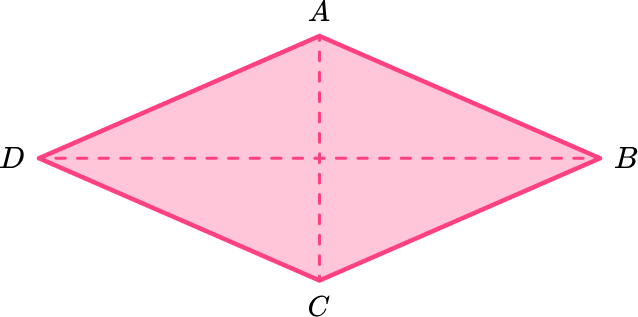6 \mathrm{~m}3 \mathrm{~m}12 \mathrm{~m}24 \mathrm{~m}The diagonals of a rhombus are perpendicular and bisect each other. They also create four congruent triangles with the same areas.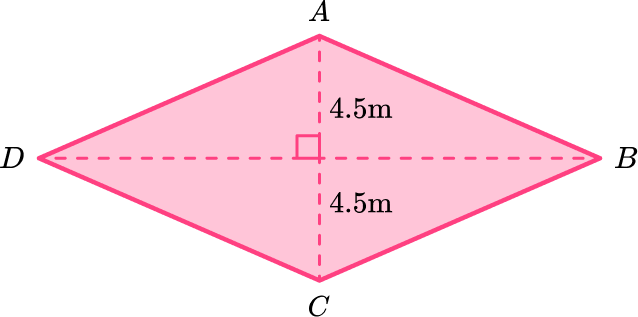Divide the area of the rhombus by 4 to find the area of one of the triangles, and then, using the area of a triangle, substitute the known values.

54 \div 4=13.5

\text{Area of triangle } =\cfrac{1}{2} \, \times base \times height

13.5=\cfrac{1}{2} \times b \times 4.5

13.5=b \times 2.25

13.5 \div 2.25=b \times 2.25 \div 2.25

6=b

The base of one triangle is 6 \, m which is half the length of the diagonal. So, double the length.

\begin{aligned} & 6 \times 2=12 \\\\ & B D=12 \mathrm{~m} \end{aligned}

Another strategy would be to use the formula,

\begin{aligned} & \text {Area }=\cfrac{1}{2} \times \text { diagonal } 1 \times \text { diagonal } 2 \\\\ & 54=\cfrac{1}{2} \times 9 \times \text { diagonal } 2 \\\\ & 54=4.5 \times \text { diagonal } 2 \\\\ & 12=\text { diagonal } 2 \\\\ & B D=12 \mathrm{~m} \end{aligned}

6) The area of a rhombus is 112 \mathrm{~cm}^2 and the height is 8 \mathrm{~cm}. Find the perimeter of the rhombus.

56 \mathrm{~cm}56 \mathrm{~cm}^214 \mathrm{~cm}14 \mathrm{~cm}^2Use the formula \text { Area }=\text { base } \times \text { height } and substitute the known values.

\begin{aligned} & 112=\text { base } \times 8 \\\\ & 112=b \times 8 \end{aligned}

\begin{aligned} & 112 \div 8=b \times 8 \div 8 \\\\ & 14=b \end{aligned}

The base of the rhombus is 14{~cm} which means the side lengths are 14{~cm}.

\begin{aligned} & \text {Perimeter }=14+14+14+14 \text { or } 4(14) \\\\ & \text {Perimeter }=56 \mathrm{~cm} \end{aligned}

## Area of rhombus FAQs

Do you have to use the Pythagorean theorem or trigonometry to find the area of a rhombus?

In high school, you will explore other strategies to find the area of rhombuses that focus on the diagonals creating four smaller right-angled triangles. Since you will be working with right triangles, you will most likely be applying the Pythagorean Theorem or right triangle trigonometry to find the length of the side or the length of the hypotenuse.

Do rhombuses have the same properties as parallelograms?

Yes, rhombuses are parallelograms, so they have opposite sides that are parallel and congruent, opposite angles that are congruent, adjacent angles that are supplementary, and diagonals that bisect each other. The interior angles add up to be 360 degrees.

## Still stuck?

At Third Space Learning, we specialize in helping teachers and school leaders to provide personalized math support for more of their students through high-quality, online one-on-one math tutoring delivered by subject experts.

Each week, our tutors support thousands of students who are at risk of not meeting their grade-level expectations, and help accelerate their progress and boost their confidence.